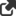# Optimal Capital Structure### ABOUT THIS CONTENT

This spreadsheet is Exhibit 1 in the Harvard Business School publication, "Note on the Theory of Optimal Capital Structure." It shows the effect of different capital structures on the stock price, expected return on equity, and other financial variables of a firm. This is the simplest possible example: the firm's EBIT is an independent random draw from the same distribution year after year, and investment exactly equals depreciation. In this situation, the market value of the firm can be computed by discounting Free Cash Flow at the Weighted Average Cost of Capital. "Optimal capital structure" means the capital structure that maximizes the stock price. This is the capital structure that maximizes the market value of the firm (debt plus equity) and the capital structure that minimizes the weighted average cost of capital. The key variables determining the optimal capital structure are the costs of debt and equity. These are simply assumed in the example.
Source: Professor Kerry Back (Washington University in St. Louis)
| visit original source   Subjects: Finance, Spreadsheets
This content is for Premium, Lifetime Access members only.
Log In Register